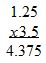# Multiplying and Dividing Decimals: Examples & Word Problems

An error occurred trying to load this video.

Try refreshing the page, or contact customer support.

Coming up next: How to Estimate with Decimals to Solve Math Problems

### You're on a roll. Keep up the good work!

Replay
Your next lesson will play in 10 seconds
• 0:01 Multiplying Decimals
• 1:35 Sales Tax: A…
• 2:41 Dividing Decimals
• 3:43 Sharing Burgers: A…
• 4:29 Lesson Summary
Save Save

Want to watch this again later?

Timeline
Autoplay
Autoplay
Speed Speed

#### Recommended Lessons and Courses for You

Lesson Transcript
Instructor: Yuanxin (Amy) Yang Alcocer

Amy has a master's degree in secondary education and has taught math at a public charter high school.

Watch this video lesson to learn what you need to do to get a correct answer when multiplying and dividing decimals. Learn the easy tricks to figuring out where the decimal point goes.

## Multiplying Decimals

Decimal numbers, those numbers with a decimal point in them, aren't scary or difficult to work with if you know the shortcuts that I will show you. In this video, we are specifically talking about multiplication and division of decimal numbers. I will show you that multiplying and dividing decimal numbers is exactly the same as multiplying and dividing whole numbers with just one difference.

In the real world, multiplying and dividing decimals happens all the time, so being able to multiply and divide decimals is an essential skill to have. For example, when you are shopping, you may need to quickly find out how much tax you can expect to pay for your purchase so that you can keep an eye out so you are not overcharged.

Let's first look at multiplying decimal numbers. Let's say we want to multiply 1.25 and 3.5. What do we do? We proceed by first multiplying the two numbers while ignoring the decimal. So we go ahead and multiply 125 with 35. We get 4,375.

Now this is where the one difference comes in and where we can apply our trick. The trick here is to count the number of decimal places we have in total. We have two from 1.25 and we have one from 3.5. We have a total of three decimal places, so that tells us that we need to count three decimal places, and that is where we put our decimal point. So our decimal goes between the 4 and the 3. Our final answer is 4.375.## Sales Tax: A Multiplication Example

Now let's look at a real-world example. You are shopping, and you want to buy two pairs of shoes. The total for the two shoes is \$62.18. The sales tax in your area is 6 percent. How much should you expect to pay in sales tax?

To solve this problem, you need to multiply your total of \$62.18 with the sales tax of 0.06 percent. Yes, we are multiplying the two decimals together. Remember what we just learned? First, go ahead and multiply as if there are no decimals. We multiply 6,218 with 6 to get 37,308. Then we count the total number of decimal places we have between our two numbers. We have four.

Now we count four decimal places in our answer to find where we should put our decimal point. We place it between the beginning 3 and the 7. Our final answer is 3.7308. We can expect to pay around \$3.73 in sales tax.

## Dividing Decimals

Let's move onto division now. Division is also similar to dividing whole numbers. The only difference here is that if the number we are dividing by is a decimal, then we will want to convert it to a whole number before we divide. If we are dividing 7.24 by 0.2, we would first change the 0.2 to a 2. To do that, we move the decimal place over one space to the right.

To unlock this lesson you must be a Study.com Member.

### Register to view this lesson

Are you a student or a teacher?

#### See for yourself why 30 million people use Study.com

##### Become a Study.com member and start learning now.
Back
What teachers are saying about Study.com

### Earning College Credit

Did you know… We have over 200 college courses that prepare you to earn credit by exam that is accepted by over 1,500 colleges and universities. You can test out of the first two years of college and save thousands off your degree. Anyone can earn credit-by-exam regardless of age or education level.